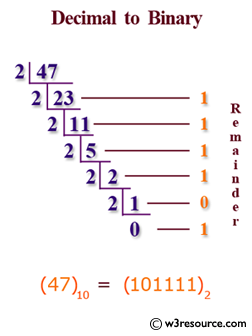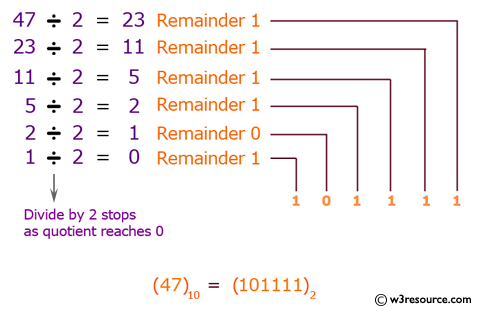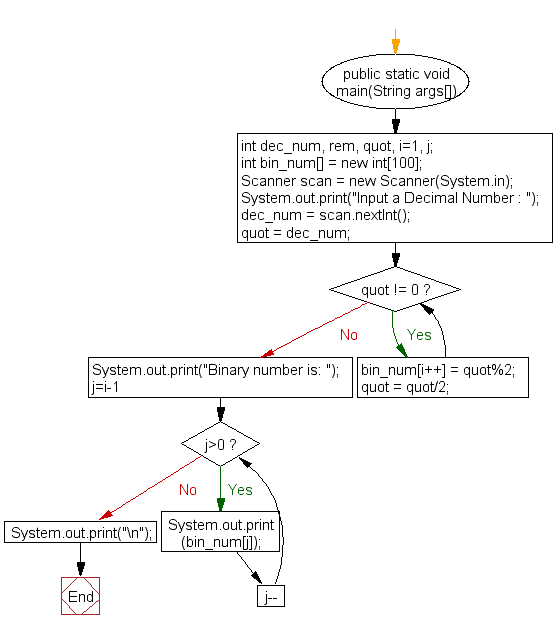﻿ Java exercises: Convert a decimal number to binary numbers - w3resource# Java Exercises: Convert a decimal number to binary numbers

## Java Basic: Exercise-19 with Solution

Write a Java program to convert a decimal number to binary number.

Decimal number: The decimal numeral system is the standard system for denoting integer and non-integer numbers. It is also called base-ten positional numeral system.

Binary number: In digital electronics and mathematics, a binary number is a number expressed in the base-2 numeral system or binary numeral system. This system uses only two symbols: typically 1 (one) and 0 (zero).

Test Data:
Input a Decimal Number : 5

Pictorial Presentation: of decimal to binary numberSample Solution:

Java Code:

``````import java.util.Scanner;
public class Exercise19 {
public static void main(String args[])
{
int dec_num, quot, i=1, j;
int bin_num[] = new int;
Scanner scan = new Scanner(System.in);

System.out.print("Input a Decimal Number : ");
dec_num = scan.nextInt();

quot = dec_num;

while(quot != 0)
{
bin_num[i++] = quot%2;
quot = quot/2;
}

System.out.print("Binary number is: ");
for(j=i-1; j>0; j--)
{
System.out.print(bin_num[j]);
}
System.out.print("\n");
}
}
```
```

Sample Output:

```Input a Decimal Number : 5
Binary number is: 101
```

Flowchart:Java Code Editor:

What is the difficulty level of this exercise?

Test your Programming skills with w3resource's quiz.

﻿

## Java: Tips of the Day

countOccurrences

Counts the occurrences of a value in an array.

Use Arrays.stream().filter().count() to count total number of values that equals the specified value.

```public static long countOccurrences(int[] numbers, int value) {
return Arrays.stream(numbers)
.filter(number -> number == value)
.count();
}
```

Ref: https://bit.ly/3kCAgLb